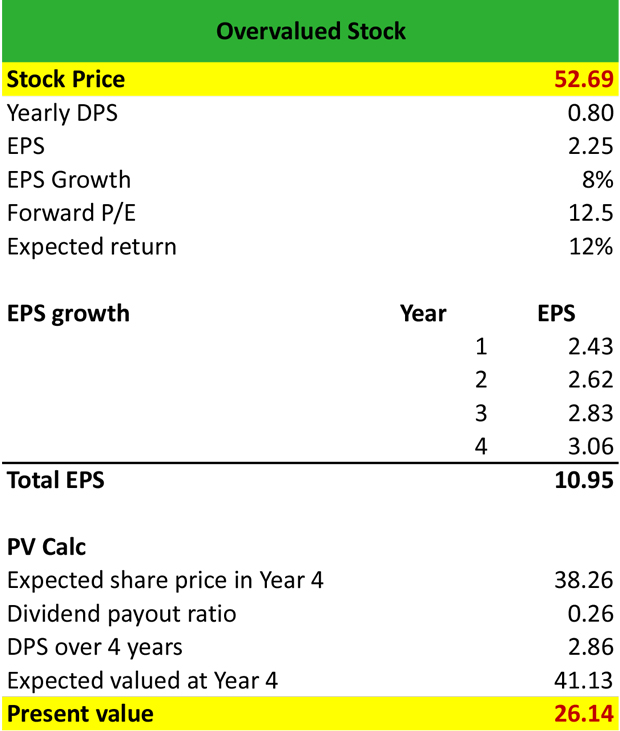# What is an Overvalued Stock?

Definition: Overvalued stocks are securities that trade higher than their fair market value, i.e. the value that the company’s fundamentals, such as earnings or revenues justify. Normally, overvalued securities are good “sell” opportunities.

## What Does Overvalued Stock Mean?

What is the definition of overvalued stock? Overvalued stocks trade at a market price that is significantly higher than their fair value (market value > fair value) as a result of increasing investor confidence or biased consensus estimates. Financial analysts use the price-to-earnings ratio (P/E) or calculate the growth rate of a firm to determine if a shareis overvalued.

Stocks are deemed as overvalued either following a surge in demand driven by rising investor confidence or if the firm’s fundamentals decline rapidly while the market price remains constant. In both cases, if the company’s fundamentals and the analyst growth projections do not justify a rise in the market price, the stock is possibly overvalued.

Let’s look at an example.

## Example

Michael is a financial analyst at Schroders. He is asked to work on a portfolio of stocks and determine if particular stocks in the portfolio possibly trade higher than their fair value.

Michael knows that the stock currently trades at \$52.69, declares an annual dividend per share of \$0.80, and has an EPS of \$2.25. He assumes an EPS growth of 8% for the next 4 years annually, a forward P/E of 12.5 and an expected return of 12%.

First, he calculates the total EPS for the next 4 years as follows:

Year 1 = EPS in Year 0 x (1 + EPS growth) = \$2.25 x (1+8%) = \$2.43

Year 2 = EPS in Year 1 x (1 + EPS growth) = \$2.43 x (1+8%) = \$2.62

Year 3 = EPS in Year 2 x (1 + EPS growth) = \$2.62 x (1+8%) = \$2.83

Year 4 = EPS in Year 3 x (1 + EPS growth) = \$2.83 x (1+8%) =\$ 3.06

The total EPS for the next 4 years is \$2.43 + \$2.62 + \$2.83 + \$3.06 = \$10.95

Then, he calculates the expected value of the stock in Year 4 as follows:

Expected share price in year 4 = EPS in year 4 x Forward P/E = \$3.06 x 12.5 = \$38.26

Dividend payout ratio = DPS / EPS in year 4 = \$0.80 / \$3.06 = 0.26

Dividend per share in Year 4 = Dividend payout ratio x total EPS = 0.26 x \$10.94 = \$2.86

Expected value at Year 4 = Expected share price in year 4 + Dividend per share in Year 4 = \$38.26 + \$2.86 = \$41.13Then, he discounts the present value of the stock by the expected return of 12% = \$41.13 x (1+12%)^4 = \$26.14

Since the stock currently trades at \$52.69 and its fair value is \$26.14, i.e. market value > fair value = the stock is overvalued.

## Summary Definition

Define Overvalued Stock: Over-valued stock means a security that isn’t worth what people are willing to pay for it.